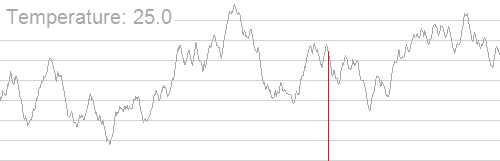## 本篇模拟退火保证 AC 率 （只要你不是只运气差到极致）

### STARTsrand(time(0));
srand(rand());
srand(rand());

while ((double)clock()/ CLOCKS_PER_SEC < 0.7)  SA();//0.7~= 700ms
// 在你程序快超时为止一直运行 SA（），注意根据你程序的快慢 修改 0.7；


## 如何生成新解

• 坐标系内：随机生成一个点，或者生成一个向量。
• 序列问题：$random\_shuffle$或者随机交换两个数。
• 网格问题：可以看做二维序列，每次交换两个格子即可。

## First ：题解 P1337【[JSOI2004] 平衡点 / 吊打 XXX】

#include <iostream>
#include <cstdio>
#include <cstring>
#include <cmath>
#include <algorithm>
#include <ctime>
#include <cstdlib>//必备头文件
using namespace std;
#define fors(i,a,b) for(int i=(a);i<=(b);++i)
typedef long long ll;
const int Size=1<<4;
inline char getch(){
static char buf[Size],*p1=buf,*p2=buf;
return p1==p2 && (p2=(p1=buf)+fread(buf,1,Size,stdin),p1==p2) ? EOF : *p1++;
}
int s=0,f=1;
char c=getch();
while(c>'9' || c<'0'){if(c=='-') f=-1;c=getch();}
while(c<='9' && c>='0'){s=(s<<1)+(s<<3)+c-'0';c=getch();}
return s*f;
}
void write(int x){
if(x<0){putchar('-');x=-x;}
if(x>9) write(x/10);
putchar(x%10+'0');
}
#define max(x,y) ((x) > (y) ? (x) : (y))
#define min(x,y) ((x) < (y) ? (x) : (y))
const int maxn=1e5+7;

struct  node
{
int x,y,w;
}a[maxn];
//以上皆为卡常
int n,sx,sy;
double ansx,ansy;

double ans=1e18,t;//解的范围

double delta=0.997;

double cal(double x,double y){
double rt=0;
fors(i,1,n){
double tmpx=x-a[i].x,tmpy=y-a[i].y;
rt+=sqrt(tmpx*tmpx + tmpy*tmpy)*a[i].w;//计算重力势能
}
return rt;
}

void SA(){
t=2500;
double x=ansx,y=ansy;
while(t>1e-14){
double tmpx=x+((rand()<<1)-RAND_MAX)*t;
double tmpy=y+((rand()<<1)-RAND_MAX)*t;//创造新解
double now=cal(tmpx,tmpy),flag=now-ans;
if(flag < 0){//如果差值小于零 ，OK
ans=now,ansx=tmpx,ansy=tmpy;
}
else if(exp(-flag/t)*RAND_MAX > rand())//以一个概率修改
x=tmpx,y=tmpy;
t*=delta;//修改温度
}
}
void AC(){
ansx=(double)sx/n,ansy=(double)sy/n;//平均值更接近正解
while((double)clock()/CLOCKS_PER_SEC < 0.83) SA();//直到你快 T 了为止一直运行
}
int main(){
srand(233333);
srand(rand());//玄学的 srand
fors(i,1,n){
sx+=a[i].x,sy+=a[i].y;//累加
}
AC();
printf("%.3lf %.3lf",ansx,ansy);
return 0;
}


## 【题解】LUOGU UVA10228 A Star not a Tree?

### 题意：给定一个 N 边形所有顶点坐标 x,y，求其费马点到所有顶点距离和

#include <iostream>
#include <cstdio>
#include <cstring>
#include <cmath>
#include <algorithm>
#include <queue>
#include <ctime>
#include <cstdlib>
using namespace std;

#define fors(i,a,b) for(int i=(a);i1e-6){
int n;
double xx,yy,ans,t;
struct point{
double x,y;
}p;
double sqr(double x){
return x*x;
}
double dis(double x,double y,point p){
return sqrt(sqr(x-p.x)+sqr(y-p.y));
}
double cal(double x,double y){
double tmp=0;
fors(i,1,n)
tmp+=dis(x,y,p[i]);
return tmp;
}
void SA(){
double tmp,x,y;
while(t>1e-6){
x=y=0;
fors(i,1,n){
x+=(p[i].x-xx)/dis(xx,yy,p[i]);
y+=(p[i].y-yy)/dis(xx,yy,p[i]);
}//在范围内构造新参数
tmp=cal(xx+x*t,yy+y*t);
if(tmp<ans){
ans=tmp;xx+=x*t,yy+=y*t;
}
else if(exp(-(tmp-ans)/t)*RAND_MAX > rand()){
ans=tmp;xx+=x*t,yy+=y*t;
}
t*=0.993;
}
}
int main()
{
srand(time(0));
srand(rand());
int ks;
cin>>ks;
fors(lk,1,ks){
cin>>n;
memset(p,0,sizeof p);
xx=yy=0;ans=1e20;t=1e6;
fors(i,1,n){
scanf("%lf%lf",&p[i].x,&p[i].y);
xx+=p[i].x;yy+=p[i].y;//累加 xx,yy
}
xx/=n;yy/=n;//为了从平均解开始
ans=cal(xx,yy);
SA();//先开始运行一次
while((double)clock()/CLOCKS_PER_SEC < (1.0/(ks+1))) SA();
printf("%.0lf",ans);
if (lk!=ks) printf("\n");
}
return 0;
}


#### B_Z_B_Y

Let us go go go！！！！！！ 　☆ *　. 　☆ 　　. ∧＿∧　∩　* ☆ * ☆ ( ・∀・)/ . 　. ⊂　　 ノ* ☆ ☆ * (つ ノ .☆ 　　 (ノ

### 4 条评论# 请阅读这篇文章的同学注意一下哦#### CraZYali · 2018年11月5日 9:55 下午

OrzOrz 大佬劲啊 Orz